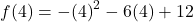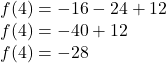Question

Evaluate the function. f(x)=−x^2 −6x+12 Find f(4)

1.thienhuong

1. How to find f(4)?

• I explained how to find f(5) in your previous question. It is exactly the same. We substitute x = 4 in the equation.

2. Substitution and EvaluateFollow BODMAS/PEMDAS rules as well! Exponent first.